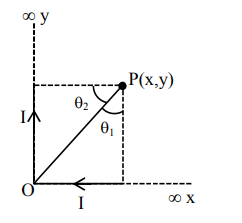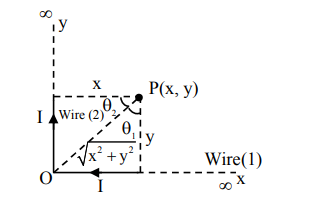# There are two infinitely long straight current carrying conductors

Question:

There are two infinitely long straight current carrying conductors and they are held at right angles to each other so that their common ends meet at the origin as shown in the figure given below. The ratio of current in both conductor is 1 : 1. The magnetic field at point P is ____.1. $\frac{\mu_{0} I}{4 \pi x y}\left[\sqrt{x^{2}+y^{2}}+(x+y)\right]$

2. $\frac{\mu_{0} I}{4 \pi x y}\left[\sqrt{x^{2}+y^{2}}-(x+y)\right]$

3. $\frac{\mu_{0} \mathrm{Ixy}}{4 \pi}\left[\sqrt{\mathrm{x}^{2}+\mathrm{y}^{2}}-(\mathrm{x}+\mathrm{y})\right]$

4. $\frac{\mu_{0} \mathrm{Ixy}}{4 \pi}\left[\sqrt{\mathrm{x}^{2}+\mathrm{y}^{2}}+(\mathrm{x}+\mathrm{y})\right]$

Correct Option: 1

Solution:$\mathrm{B}_{\text {due to wire (1) }}=\frac{\mu_{0} \mathrm{I}}{4 \pi \mathrm{y}}\left[\sin 90+\sin \theta_{1}\right]$

$=\frac{\mu_{0}}{4 \pi} \frac{\mathrm{I}}{\mathrm{y}}\left(1+\frac{\mathrm{x}}{\sqrt{\mathrm{x}^{2}+\mathrm{y}^{2}}}\right) \ldots \ldots(1)$

$\mathrm{B}_{\text {due to wire (2) }}=\frac{\mu_{0}}{4 \pi} \frac{\mathrm{I}}{\mathrm{x}}\left(\sin 90^{\circ}+\sin \theta_{2}\right)$

$=\frac{\mu_{0}}{4 \pi} \frac{\mathrm{I}}{\mathrm{x}}\left(1+\frac{\mathrm{y}}{\sqrt{\mathrm{x}^{2}+\mathrm{y}^{2}}}\right) \ldots \ldots(2)$

Total magnetic field

$\mathrm{B}=\mathrm{B}_{1}+\mathrm{B}_{2}$

$\mathrm{B}=\frac{\mu_{0} \mathrm{I}}{4 \pi}\left[\frac{1}{\mathrm{y}}+\frac{\mathrm{x}}{\mathrm{y} \sqrt{\mathrm{x}^{2}+\mathrm{y}^{2}}}+\frac{1}{\mathrm{x}}+\frac{\mathrm{y}}{\mathrm{x} \sqrt{\mathrm{x}^{2}+\mathrm{y}^{2}}}\right]$

$B=\frac{\mu_{0} \mathrm{I}}{4 \pi}\left[\frac{x+y}{x y}+\frac{x^{2}+y^{2}}{x y \sqrt{x^{2}+y^{2}}}\right]$

$B=\frac{\mu_{0} I}{4 \pi}\left[\frac{x+y}{x y}+\frac{\sqrt{x^{2}+y^{2}}}{x y}\right]$

$B=\frac{\mu_{0} I}{4 \pi x y}\left[\sqrt{x^{2}+y^{2}}+(x+y)\right]$

Option (1)